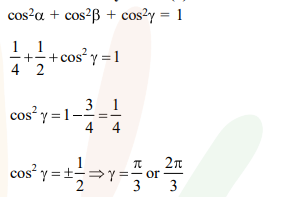# Solve this following`
Question:

If a unit vector $\overrightarrow{\mathrm{a}}$ makes angles $\pi / 3$ with $\hat{\mathrm{i}}, \pi / 4$ with $\hat{\mathrm{j}}$ and $\theta \in(0, \pi)$ with $\hat{\mathrm{k}}$, then a value of $\theta$ is :-

1. $\frac{5 \pi}{12}$

2. $\frac{5 \pi}{6}$

3. $\frac{2 \pi}{3}$

4. $\frac{\pi}{4}$

Correct Option: , 3

Solution: